## MP Board Class 6th Maths Solutions Chapter 6 Integers Ex 6.1

Question 1.
Write opposites of the following :
(a) Increase in weight
(b) 30 km north
(c) 326 BC
(d) Loss of ₹ 700
(e) 100 m above sea level
Solution:
(a) Decrease in weight
(b) 30 km south
(d) Profit of ₹ 700
(e) 100 m below sea level

Question 2.
Represent the following numbers as integers with appropriate signs.
(a) An aeroplane is flying at a height, two thousand metre above the ground.
(b) A submarine is moving at a depth, eight hundred metre below the sea level.
(c) A deposit of rupees two hundred.
(d) Withdrawal of rupees seven hundred.
Solution:
(a) Two thousand metre above the ground = + 2000 metres
(b) Eight hundred metre below the sea level = – 800 metres
(c) Deposit of two hundred rupees = + 200 Rupees
(d) Withdrawal of seven hundred rupees = -700 Rupees

Question 3.
Represent the following numbers on a number line:
(a) +5
(b) -10
(c) + 8
(d) -1
(e) -6
Solution: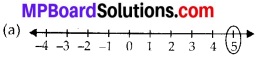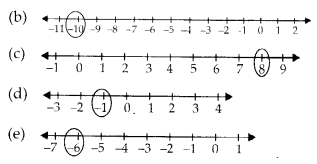Question 4.
Adjacent figure is a vertical number line, representing integers. Observe it and locate the following points :
(a) If point D is + 8, then which point is-8?
(b) Is point G a negative integer or a positive integer?
(c) Write integers for points B and E.
(d) Which point marked on this number line has the least value?
(e) Arrange all the points in decreasing order of value.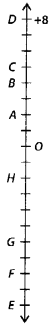Solution:
(a) We have, point D is +8. Therefore, 16 steps to the down from D is -8 i.e., the point F.
(b) Point G is a negative integer.
(c) Point B is four steps down from point D.
∴ Value of point B = +8 – 4 = +4
Point E is eighteen steps down from point D.
∴ Value of point E = +8 – 18 = -10
(d) Since, point E is located in the bottom. So, point E has the least value.
(e) Decreasing order of all the points is, D, C, B, A, O, H, G, F, EQuestion 5.
Following is the list of temperatures of five places in India on a particular day of the year.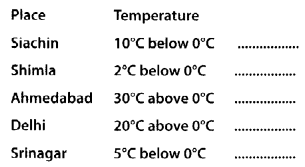(a) Write the temperatures of these places in the form of integers in the blank column.
(b) Following is the number line representing the temperature in degree Celsius.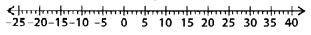Plot the name of the city against its temperature.
(c) Which is the coolest place?
(d) Write the names of the places where temperatures are above 10°C.
Solution: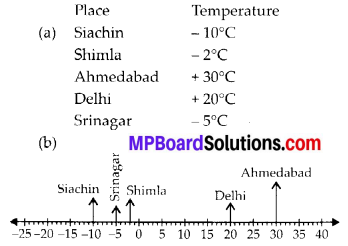(c) Siachin is the coolest place.
(d) Ahmedabad and Delhi have temperature above 10°C.

Question 6.
In each of the following pairs, which number is to the right of the other on the number line?
(a) 2, 9
(b) -3, -8
(c) 0, -1
(d) -11, 10
(e) – 6, 6
(f) 1,-100
Solution:
(a) 9 is right to 2
(b) -3 is right to -8
(c) 0 is right to -1
(d) 10 is right to -11
(e) 6 is right to -6
(f) 1 is right to-100

Question 7.
Write all the integers between the given pairs (write them in the increasing order.)
(a) 0 and -7
(b) -4 and 4
(c) – 8 and – 15
(d) -30 and -23
(a) The integers between 0 and -7 are -6, -5, -4, -3, -2, -1
(b) The integers between -4 and 4 are -3, -2, -1, 0, 1, 2, 3
(c) The integers between -8 and -15 are -14, -13, -12,-11,-10, -9
(d) The integers between -30 and -23 are -29, -28, -27, -26, -25, -24

Question 8.
(a) Write four negative integers greater than -20.
(b) Write four negative integers less than -10.
Solution:
(a) There are 19 negative integers which are greater than -20. Four of them are -19, -18, -17, -16
(b) There are infinite negative integers which are less than -10. Four of them are -11, -12, -13, -14

Question 9.
For the following statements, write True (T) or False (F). If the statement is false, correct the statement.
(a) – 8 is to the right of – 10 on a number line.
(b) – 100 is to the right of – 50 on a number line.
(c) Smallest negative integer is – 1.
(d) – 26 is greater than – 25.
Solution:
(a) True
(b) False
Since -100 is to the left of -50 on the number line.
(c) False
Since -1 is the greatest negative integer.
(d) False
Since -26 is less than -25.Question 10.
Draw a number line and answer the following :
(a) Which number will we reach if we move 4 numbers to the right of – 2.
(b) Which number will we reach if we move 5 numbers to the left of 1.
(c) If we are at – 8 on the number line, in which direction should we move to reach – 13?
(d) If we are at – 6 on the number line, in which direction should we move to reach – 1 ?
Solution: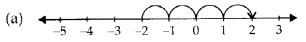Thus, we will reach 2 if we move 4 numbers to the right of -2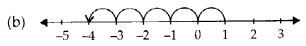Thus, we will reach -4 if we move 5 numbers to the left of 1.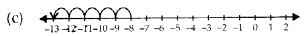Thus, we should move 5 numbers to the left of -8 to reach -13.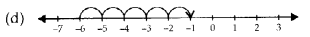Thus, we should move 5 numbers to the right of -6 to reach -1.